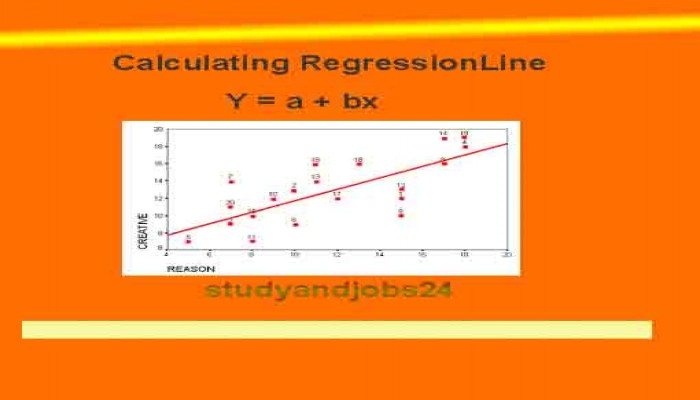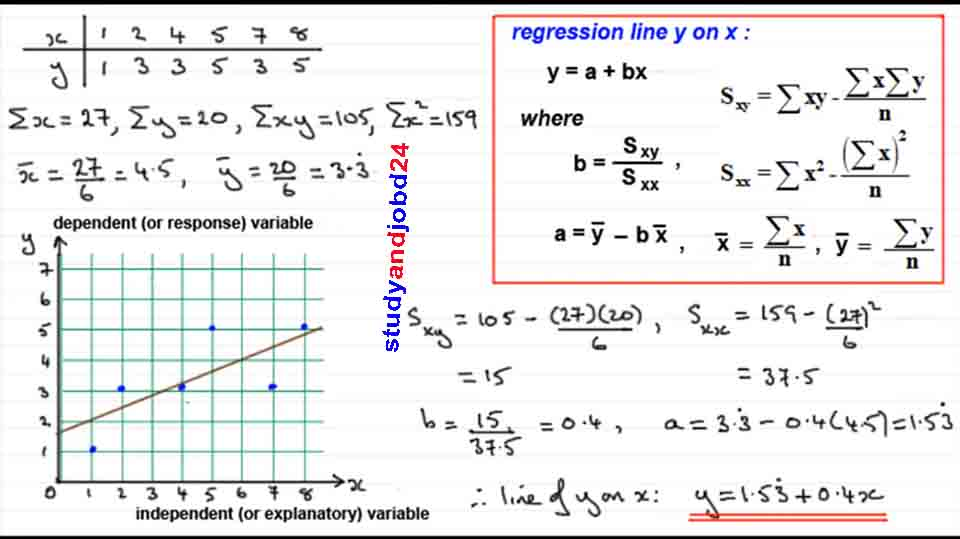Regression Equation: Y = a + bx

A linear regression line has an equation of the form Y = a + bX, where X is the explanatory variable and Y is the dependent variable. The slope of the line is b, and a is the intercept (the value of y when x = 0)

Where,

,

and

Problem:

Find the Regression Equation and Line from the following data

 X 1 2 4 5 7 8 Y 1 3 3 5 3 5

Solutions:

andPlease share this post and visit our facebook page to get more.